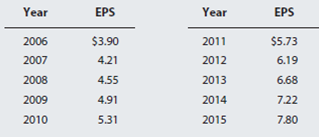Chapter 10, Problem 20PFundamentals of Financial Manageme...

14th Edition
Eugene F. Brigham + 1 other
ISBN: 9781285867977

Solutions

Chapter
SectionFundamentals of Financial Manageme...

14th Edition
Eugene F. Brigham + 1 other
ISBN: 9781285867977
Textbook Problem

WACC The following table gives Foust Company’s earnings per share for the last 10 years. The common stock, 7 8 million shares outstanding, is now (1/1/16) selling for $65 00 per share. The expected dividend at the end of the current year (12/31/16) is 55% of the 2015 EPS. Because investors expect past trends to continue, g may be based on the historical earnings growth rate. (Note that 9 years of growth are reflected in the 10 years of data.The current interest rate on new debt is 9%; Foust’s marginal tax rate is 40%; and its target capital structure is 40% debt and 60% equity. a. Calculate Foust’s after-tax cost of debt and common equity. Calculate the cost of equity as rs = D1 P0 + g. b. Find Foust’s WACC. a. Summary Introduction To identify: The after-tax cost of debt and cost of common equity. Introduction: Cost of Capital: In any company there is a need of the fund for various purposes. The companies raise capital through various sources such as equity, debt, and preferred stock. The cost of capital is the total cost of raising capital. It consists of the cost of debt, cost of equity, and cost of preferred stock. After-Tax Cost of Debt It can be defined as a relevant cost of the new debt by considering the tax deductibility in interest. It is the cost of the debt after tax savings. The interest on the debt is tax deductible. The tax can saved on the interest paid on the debt. It is used to calculate the weighted average cost of capital. Cost of Equity It is the cost of the company while raising finance by issuing equity. It is the earnings from the investment to the firm’s equity investors. It is the return to the stockholders’ equity investments. The issue of new stock incurs the flotation cost. Explanation After-tax cost of debt Given, Before-tax cost of debt is 9%. Tax rate is 40%. The formula to calculate after-tax cost of debt is: After-Tax Cost of Debt=rd(1t) Where • rd is the interest on new debt. • t is the tax rate Substitute 9% for rd and 0.40 for the t in above formula. After-Tax Cost of Debt=9%(10.40)=9%×0.60=5.4% Thus, after-tax cost of debt is 5.4%. Cost of equity Given, Expected dividend, D1 is$4.29($7.8×55%). Current market price P0 is$65 per share.

Growth rate is 8% or 0.08.

The formula to calculate cost of the equity is:

r=D1P0+g

Where,

• D1 is the next expected dividend.
• P0 is the current market price.
• g is the growth rate.

Substitute $4.29 for D1,$65 for P0, 0

b.

Summary Introduction

To determine: WACC of F Company.

Introduction:

Weighted Average Cost of Capital

It is the weighted average cost of capital of all the sources through which the firm finances its capital. It is the rate that a company will pay to all for raising finance. It can be termed as the firm’s cost of capital. The company raises money through various sources such as common stock and preference share debt. The WACC is calculated by taking the relative weight of each item of capital structure.

The formula of WACC is:

WACC=Wdrd(1t)+WPrp+Wcrs

Where

• Wd is the weight of the debt.
• WP is the weight of the preferred stock.
• Wc is the weight of the equity.
• rd is cost of the debt.
• rP is cost of the preferred stock.
• rc is the cost of the equity.

Still sussing out bartleby?

Check out a sample textbook solution.

See a sample solution

The Solution to Your Study Problems

Bartleby provides explanations to thousands of textbook problems written by our experts, many with advanced degrees!

Get Started